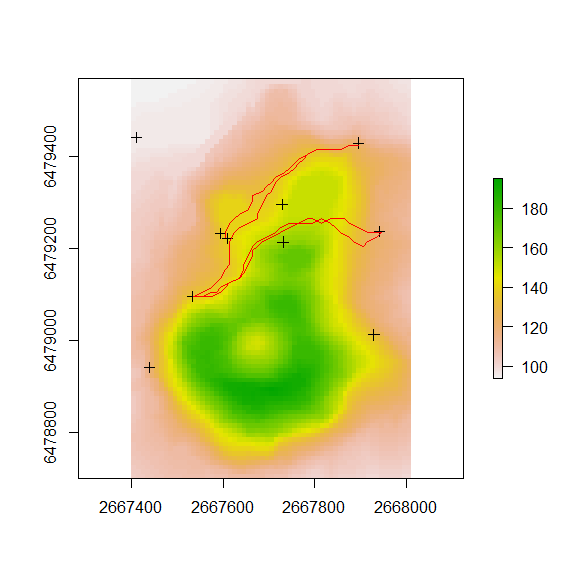# 1. Introduction

This vignette describes leastcostpath, a package written for use in the R environment (R Core Team, 2016). It provides functionality to calculate Least Cost Paths using multiple cost functions that approximate the difficulty of moving across a landscape, taking into account obstacles and local fricion (e.g. slope). Furthermore, this package allows for the incorporation of cost when traversing across slope, as well as other factors such as landscape features.

# 2. Setup

library(rgdal)
library(rgeos)
library(sp)
library(raster)
library(gdistance)
library(leastcostpath)
r <- raster::raster(system.file('external/maungawhau.grd', package = 'gdistance'))

r_extent <- as(raster::extent(r), 'SpatialPolygons')

crs(r_extent) <- crs(r)

plot(r)
plot(r_extent, add = T, border = "red")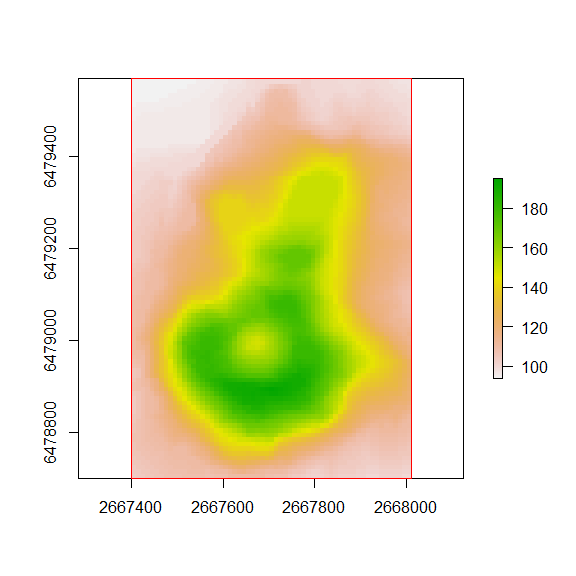# 3. Example 1: Least Cost Path Analysis (Slope only)

cs <- create_slope_cs(dem = r, cost_function = 'tobler', neighbours = 16)
loc1 = cbind(2667876, 6479424)
loc1 = sp::SpatialPoints(loc1)

loc2 = cbind(2667677, 6478737)
loc2 = sp::SpatialPoints(loc2)

lcp <- create_lcp(cost_surface = cs, origin = loc1, destination = loc2, directional = FALSE)

plot(raster(cs))
plot(lcp[1,], add = T, col = "red")
plot(lcp[2,], add = T, col = "blue")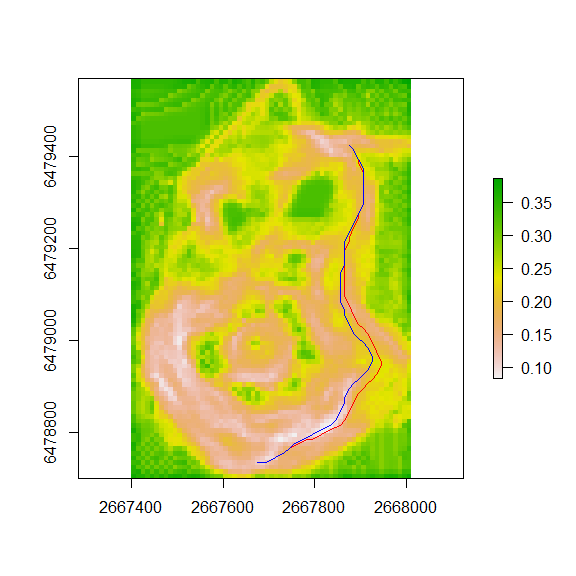# 4. Example 2: Least Cost Path Analysis (Slope and Traversal Across Slope)

cs <- create_slope_cs(dem = r, cost_function = 'tobler', neighbours = 16) %>%
"*" (create_traversal_cs(dem = r, neighbours = 16))
loc1 = cbind(2667876, 6479424)
loc1 = sp::SpatialPoints(loc1)

loc2 = cbind(2667677, 6478737)
loc2 = sp::SpatialPoints(loc2)

lcp <- create_lcp(cost_surface = cs, origin = loc1, destination = loc2, directional = FALSE)

plot(raster(cs))
plot(lcp[1,], add = T, col = "red")
plot(lcp[2,], add = T, col = "blue")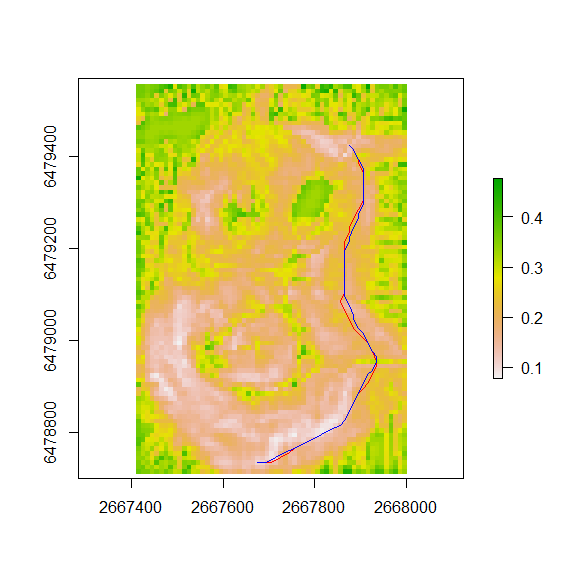# 5. Example 3: Least Cost Path Analysis (Slope, Traversal Across Slope, and Landscape Feature)

feature_loc = cbind(2667652, 6478997)
feature_loc = sp::SpatialPoints(feature_loc)

x <- seq(100, 1, length.out = 20)

cs <- create_slope_cs(dem = r, cost_function = 'tobler', neighbours = 16) %>%
"*" (create_traversal_cs(dem = r, neighbours = 16)) %>%
"*" (create_feature_cs(raster = r, locations = feature_loc, x))
## note: create_feature_cs expects planar coordinates
loc1 = cbind(2667876, 6479424)
loc1 = sp::SpatialPoints(loc1)

loc2 = cbind(2667677, 6478737)
loc2 = sp::SpatialPoints(loc2)

lcp <- create_lcp(cost_surface = cs, origin = loc1, destination = loc2, directional = FALSE)

plot(raster(cs))
plot(feature_loc, add = T, col = "black")
plot(lcp[1,], add = T, col = "red")
plot(lcp[2,], add = T, col = "blue")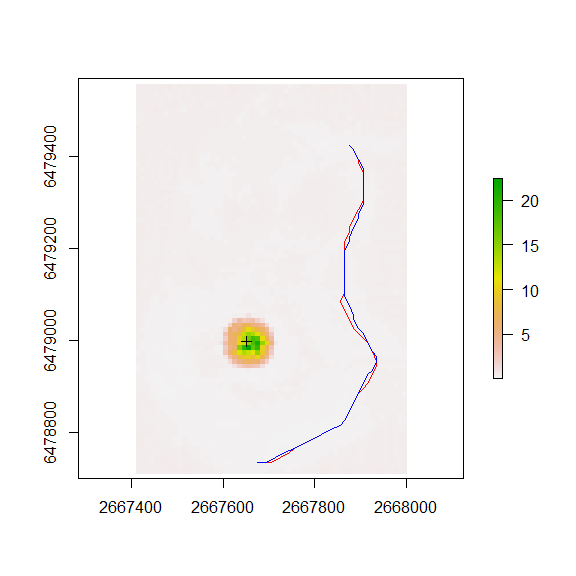# 6. Example 4: Least Cost Path Corridor (Slope, Traversal Across Slope, and Landscape Feature)

feature_loc = cbind(2667652, 6478997)
feature_loc = sp::SpatialPoints(feature_loc)

x <- seq(100, 1, length.out = 20)

cs <- create_slope_cs(dem = r, cost_function = 'tobler', neighbours = 16) %>%
"*" (create_traversal_cs(dem = r, neighbours = 16)) %>%
"*" (create_feature_cs(raster = r, locations = feature_loc, x))
## note: create_feature_cs expects planar coordinates
loc1 = cbind(2667876, 6479424)
loc1 = sp::SpatialPoints(loc1)

loc2 = cbind(2667677, 6478737)
loc2 = sp::SpatialPoints(loc2)

cc <- create_cost_corridor(cs, loc1, loc2)

plot(cc)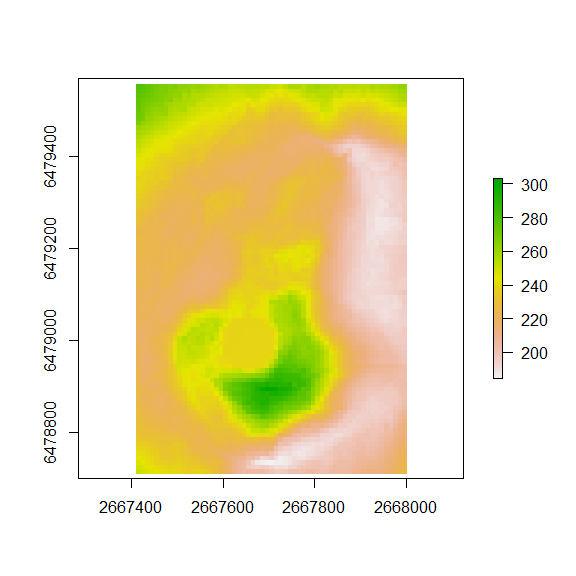# 6. Example 5: From Everywhere to Everywhere (Slope and Traversal Across Slope)

locs <- sp::spsample(r_extent,n=4,'regular')

lcp_network <- create_slope_cs(dem = r, cost_function = 'tobler', neighbours = 16) %>%
"*" (create_traversal_cs(dem = r, neighbours = 16)) %>%
create_FETE_lcps(cost_surface = ., locations = locs, cost_distance = FALSE, parallel = FALSE)

plot(r)
plot(lcp_network, add = T, col = "red")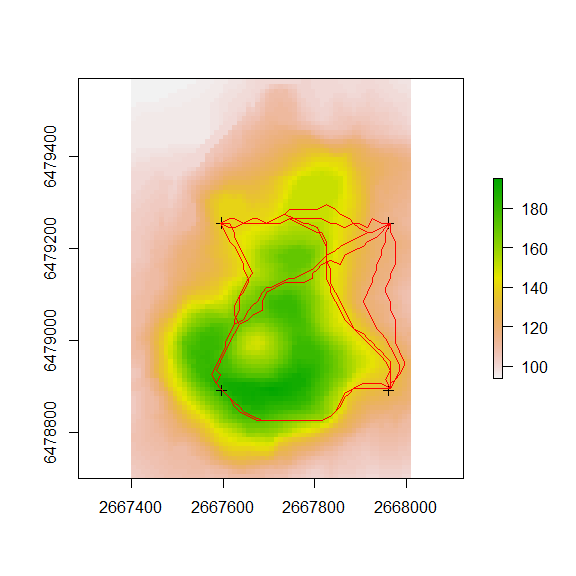# 7. Example 6: Least Cost Paths Density

lcp_network_density <- create_lcp_density(lcps = lcp_network, raster = r, rescale = TRUE)

plot(lcp_network_density)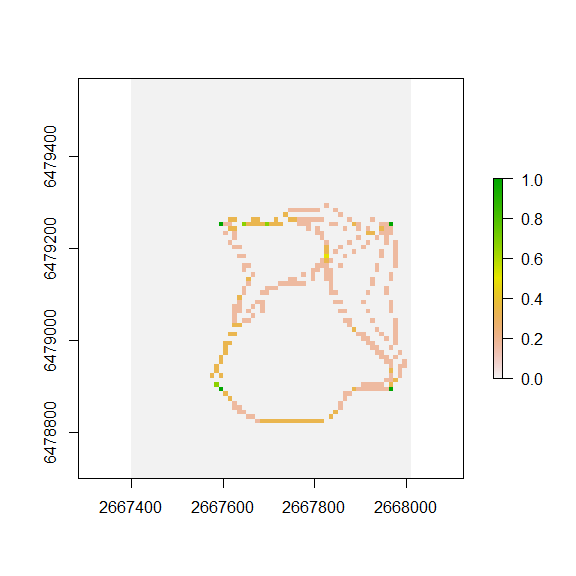# 8. Example 7: Least Cost Paths Kernel Density

fwModel <- raster::focalWeight(lcp_network_density, max(res(r)) * 2, type='circle')

fwModel[fwModel>0] <- 1

lcp_network_kd <- raster::focal(lcp_network_density, w=fwModel ,fun=sum , na.rm=TRUE)

plot(lcp_network_kd)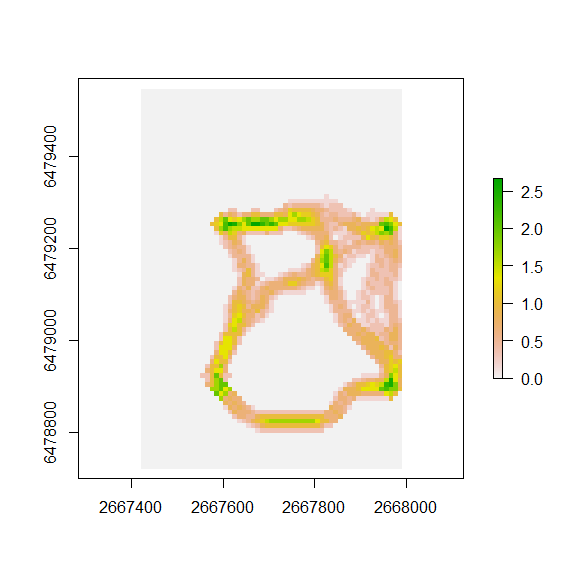# 9. Example 8: Cumulative Cost Paths from Radial Locations (Slope and Traversal Across Slope)

locs = cbind(2667652, 6478997)
locs = sp::SpatialPoints(locs)

lcp_network <- create_slope_cs(dem = r, cost_function = 'tobler', neighbours = 16) %>%
"*" (create_traversal_cs(dem = r, neighbours = 16)) %>%
create_CCP_lcps(cost_surface = ., location = locs, distance = 100, radial_points = 50, cost_distance = FALSE, parallel = FALSE)

plot(r)
plot(lcp_network, add = T, col = "red")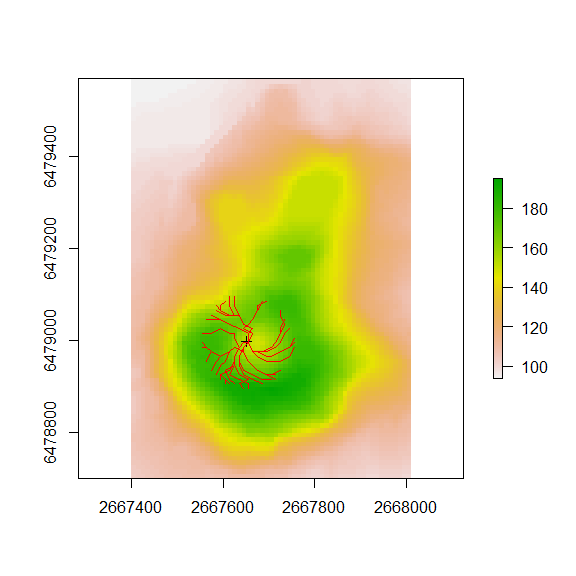# 10. Example 9: Least Cost Paths from random locations within distances (Slope and Traversal Across Slope)

locs = cbind(2667652, 6478997)
locs = sp::SpatialPoints(locs)

lcp_network <- create_slope_cs(dem = r, cost_function = 'tobler', neighbours = 16) %>%
"*" (create_traversal_cs(dem = r, neighbours = 16)) %>%
create_banded_lcps(cost_surface = ., location = locs, min_distance = 5, max_distance = 250, radial_points = 50, cost_distance = FALSE, parallel = FALSE)

plot(r)
plot(lcp_network, add = T, col = "red")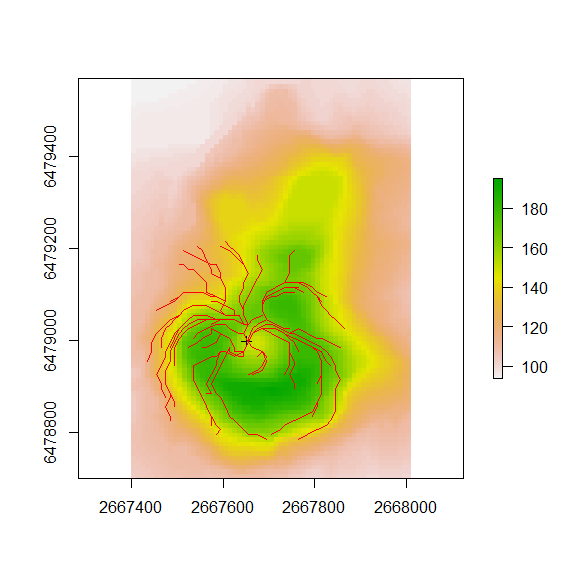# 11. Example 10: Least Cost Path Network (Slope and Traversal Across Slope)

See Least Cost Path Vignette 2 for more examples using Least Cost Path Network

locs <- sp::spsample(r_extent,n=10,'random')

lcp_network <- create_slope_cs(dem = r, cost_function = 'tobler', neighbours = 16) %>%
"*" (create_traversal_cs(dem = r, neighbours = 16)) %>%
create_lcp_network(., locations = locs, nb_matrix = cbind(c(1, 4, 2, 1), c(2, 2, 4, 3)), cost_distance = FALSE, parallel = FALSE)

plot(r)
plot(lcp_network, add = T, col = "red")